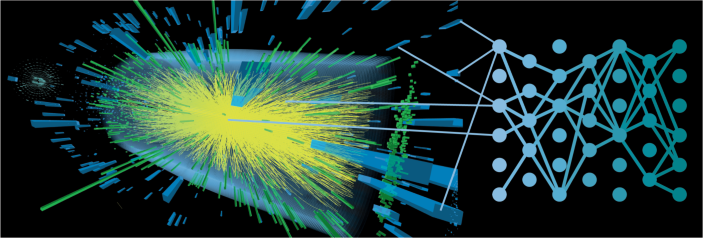To turn the vast amount of data collected in particle collider experiments into precise measurements of nature, particle physicists have to find subtle patterns in high-dimensional data. To this end, particle physicists use complex computer simulations which encode our understanding of elementary particle collisions, additional radiation patterns, particle decays, hadronization, interactions with the detector, and sensor readout. Unfortunately, the analysis of particle-collision data with computer simulations often faces a fundamental challenge.

Simulations implement a forward process: given a value of the physical parameters, they can generate synthetic observations. In the scientific process, however, physicists are interested in the inverse problem: given observed data, what is the most likely value of the physical parameters? The key quantity required for this inference, both in a frequentist and a Bayesian statistical framework, is the likelihood function, or the probability density of observed data as a function of the parameters. However, in general it is not possible to compute the likelihood function – this intractability is an essential problem for data analysis.Credit: CERN, https://cds.cern.ch/record/2646378

Over the years, different methods for parameter inference have been developed that do not require a tractable likelihood function. These methods are collectively known as simulation-based, or likelihood-free, inference techniques. Historically, the most common approach has been to reduce both observed and simulated high-dimensional data to a single kinematic variable. The likelihood function in this one-dimensional summary statistic can then be estimated with histograms, enabling both frequentist and Bayesian inference. But the reduction to a single kinematic variable almost invariably discards useful information from the original data, and parameter measurements constructed in this way will then be less precise than an analysis of the full high-dimensional data could be. The histogram method does not scale to high-dimensional data as it would require an exorbitantly large number of simulations to be run.

The impressive recent progress in machine learning models and algorithms has made possible many powerful new simulation-based inference techniques, which enable the precise parameter measurements directly from the high-dimensional data. In a nutshell, these methods proceed by training a machine learning model, such as a neural network, on data from the simulator. During the inference step, the model then acts as a surrogate for the computer simulations. Further tweaks can improve the efficiency of this approach: the number of required simulation runs can be reduced with active learning methods, which iteratively steer simulations to the most promising settings given past results. Moreover, some of these methods tightly integrate simulation and inference, which can often further improve sample efficiency and the quality of inference.

On the one hand, simulation-based inference is universal. These techniques have been used to solve problems in various fields of science. The domain-agnostic statistical framing allows scientists from different areas, statisticians, and computer scientists to develop a rich methodology together and to learn from each other. On the other hand, simulation-based inference methods can be tailored to the structure of each particular problem. In particle physics, for example, latent variables that are useful in some inference methods are deeply connected to the matrix elements that govern the elementary particle interactions and are based on quantum field theory. One can use this link and the understanding of the structure of particle physics processes to make data analysis for particle physics particularly efficient. These methods are automated in MadMiner, an open-source Python library that wraps around standard Large Hadron Collider simulators and machine learning libraries.

Simulation-based inference methods have so far been applied in phenomenological studies to precision measurements of the Higgs boson, to searches for indirect effects of new physics in effective field theories, and in the search for direct signals of heavy new particles. In all cases, the new machine learning–based techniques led to more a sensitive analysis than the traditional approach. Further progress in machine learning, the development of new inference algorithms, together with the continued development of tools such as MadMiner will only improve the capabilities of data analysis. I am excited to see how these new simulation-based inference methods will be used to analyze data collected at the Large Hadron Collider experiments.